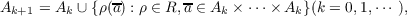#### Vol. 53, No. 2, 1974

 Download this articleFor screen For printingRecent Issues Vol. 307: 1  2 Vol. 306: 1  2 Vol. 305: 1  2 Vol. 304: 1  2 Vol. 303: 1  2 Vol. 302: 1  2 Vol. 301: 1  2 Vol. 300: 1  2Online Archive Volume: Issue:The Journal Editorial Board Subscriptions Officers Special Issues Submission Guidelines Submission Form Contacts ISSN: 1945-5844 (e-only) ISSN: 0030-8730 (print) Author Index To Appear Other MSP Journals
Arithmetic properties of certain recursively defined sets

### David Anthony Klarner and R. Rado

Vol. 53 (1974), No. 2, 445–463
##### Abstract

Let R denote a set of linear operations defined on the set N of nonnegative integers; for example, a typical element of R has t he form ρ(x1,,xr) = m0 + m1x1 + + mrxr where m0,,mr denote certain integers. Given a set A of positive integers, there is a smallest set of positive integers denoted R : Awhich contains A as a subset and is closed under every operation in R. The set R : Acan be constructed recursively as follows: Let A0 = A, and definethen it can be shown that R : A= A0 A1 . The sets R : Asometimes have an elegant form, for example, the set 2x + 3y : 1consists of all positive numbers congruent to 1 or 5 modulo 12. The objective is to give an arithmetic characterization of elements of a set R : A. This paper is a report on progress made on this problem when the authors collaborated at Reading University in the academic year 1970–71.

##### Mathematical Subject Classification 2000
Primary: 10A99
Secondary: 05B10, 04A05
##### Milestones